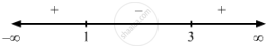PUC Karnataka Science Class 12Department of Pre-University Education, Karnataka
Share

F(X) = X3 − 6x2 + 9x + 15 . - PUC Karnataka Science Class 12 - Mathematics

Question

f(x) =  x$-$ 6x2 + 9x + 15 .

Solution

$\text { Given: } f\left( x \right) = x^3 - 6 x^2 + 9x + 15$

$\Rightarrow f'\left( x \right) = 3 x^2 - 12x + 9$

$\text { For a local maximum or a local minimum, we must have }$

$f'\left( x \right) = 0$

$\Rightarrow 3 x^2 - 12x + 9 = 0$

$\Rightarrow x^2 - 4x + 3 = 0$

$\Rightarrow \left( x - 1 \right)\left( x - 3 \right) = 0$

$\Rightarrow x = 1 \text { or } 3$Sincef '(x) changes from negative to positive when x increases through 3, x = 3 is the point of local minima.

The local minimum value of  f (x) at x = 3 is given by $\left( 3 \right)^3 - 6 \left( 3 \right)^2 + 9\left( 3 \right) + 15 = 27 - 54 + 27 + 15 = 15$

Since f '(x) changes from positive to negative when x increases through 1, x = 1 is the point of local maxima.

The local maximum value of  f (x) at x = 1 is given by $\left( 1 \right)^3 - 6 \left( 1 \right)^2 + 9\left( 1 \right) + 15 = 1 - 6 + 9 + 15 = 19$
Is there an error in this question or solution?

Video TutorialsVIEW ALL 

Solution F(X) = X3 − 6x2 + 9x + 15 . Concept: Graph of Maxima and Minima.
S